Electricity & Megnetism

# Electrical force

Electric force is the force that one electric charge exerts on another. It is repulsive for charges of the same sign and attractive for charges of opposite signs. Furthermore, it is a vector quantity , proportional to the magnitude of the charges and inversely proportional to the distance that separates them, being mathematically described by Coulomb’s law.

This force makes atoms repel or attract each other, thus giving rise to different types of chemical bonds, for example. Understanding how electrical power works is fundamental to understanding various natural phenomena, as well as the large number of technologies that we have today that work based on electricity .

## What is electrical force?

Electric force is the interaction exerted between electric charges. Electric charges of the same sign repel each other when brought together, and electric charges of different signs are attracted. The electrical force that a charge exerts on another charge is proportional to the product of the magnitude of their charges and inversely proportional to the square of the distance separating them.

The electric force is also a vector quantity , as it has magnitude, direction and sense. The unit of measurement for electrical force is the newton. Furthermore, it is important to remember that, regardless of the magnitude of the charges that interact, the electric force exerted on both charges is equal, as a result of Newton’s third law , called the Law of Action and Reaction.

The law used to quantify the intensity of the electrical force that a charge produces on other charges is Coulomb’s law , which was named after its discoverer, French physicist Charles Augustin Coulomb .

By means of a torsion balance, created by himself, Charles Coulomb was able to accurately determine the intensity of the force between loads. Based on his experimental results, he deduced the mathematical law that explains the force of interaction between electric charges.

“The force of attraction between electric charges is proportional to the product of their charges and inversely proportional to the square of the distance that separates them.”

## Coulomb’s Law

The formula below shows the expression used to calculate the intensity of the force of attraction or repulsion between two point particles (particles of negligible dimensions) charged with electric charges of magnitude 1 and 2 , separated in vacuum by a distance d.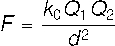1 and Q 2 – modules of electrical charges (C)

d – distance between loads (m)

0 – electrostatic vacuum constant (k 0 = 9.10 9 Nm²/C²)

Since the magnitude of the electric force that acts between two charges is inversely proportional to the distance that separates them, the graph that relates this force to the separation between the charges will have a format similar to the following graph: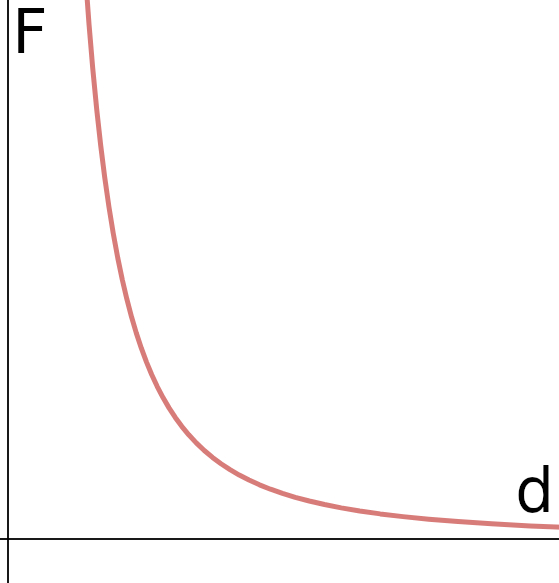## Electric force and electric field

Electric force and electric field are closely related quantities . Every electric charge produces a field around it, called an electric field . When an electrically charged particle with a positive charge is dropped into a region where there is an electric field, an electric force appears on it proportional to the magnitude of its charge. However, when a negative electric charge is released under the same conditions, it moves in the opposite direction to the direction of the electric field.

The electric field is, by definition, the electric force produced per unit charge . The relationship between the electric field and the electric force is as follows: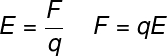E – electric field (N/C)

q – electrical charge

## electrical force work

Electric force work is the amount of energy that an electric charge “gains” or “loses” when moving along a region of nonzero electric field. For some work to be done on the electric charge, it is necessary for it to move, even partially, in the   direction of the electric field lines . The formula used to calculate the work done by the electric force is shown in the following figure:τ – work (J)

d – distance

θ – angle between F and d

Now that we have learned about what electrical force is and how to calculate it, how about learning more with solved exercises on the subject?

## Exercises solved on electrical force

Question 1 — Two identical point charges of 1.0 mC each are spaced 0.5 m apart in vacuum. Determine the magnitude of the electric force between these two charges.

a) 6.4.10 4 N

b) 3.6.10 4 N

c) 7.2.10 3 N

d) 1.2.10 5 N

Resolution:

Let’s use Coulomb’s law to calculate the magnitude of the force between the charges.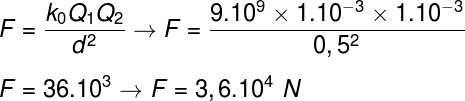Based on the result obtained above, it is concluded that the correct alternative is the letter B .

Question 2 — Two particles of electric charge Q are separated in vacuum by a distance d. Doubling this distance, the magnitude of the electric force F that one of these charges exerts on the other will be:

a) 2F

b) F/2

c) F/4

d) 4F

Resolution :

To solve the exercise, it is necessary to apply the data from the statement in Coulomb’s law.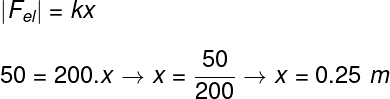Based on the result obtained, the correct alternative is the letter C .

Question 3 — An electric charge of 0.5 nC is placed in a region where there is an electric field of 500 N/m. Determine the magnitude of the electric force on this charge and mark the correct alternative:

a) 2.5.10 -7 N

b) 0.5.10 -6 N

c) 1.5.10 -5 N

d) 4.5.10 -4 N

Resolution :

To calculate the electric force acting on the charge, you need to use the formula that relates the electric force to the electric field. Doing this, just solve the following calculation: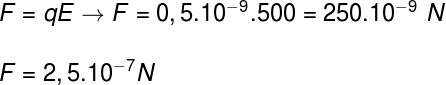The correct alternative, therefore, is the letter a .